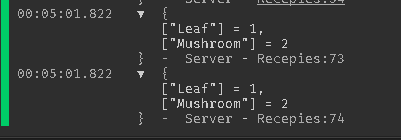# Help with Tables and Potion System

I’d like to be able to find out if a Table has the same Values as Another table, for a check.

I have no idea how to do it and I have found no answers yet to roblox studio.On the first Table is whats inside the Cauldren, and on the Second one is what the Cauldren should have inside for it to create this:It would be amazing if you guys could help me!

``````local Recepies = {
["Ingredients"] = {
["Mushroom"] = {
Color = Color3.fromRGB(130, 0, 0);
};
["Leaf"] = {
Color = Color3.fromRGB(0, 130, 30);
};
["e"] = {
Color = Color3.fromRGB(0, 130, 30);
};
};

["Outcomes"] = {
["Potion"] = {
["Mushroom"] = 2;
["Leaf"] = 1;
};
};

["Tables"] = {}
}

local Cauldren = workspace.Cauldren[cauldrenName]
local Table

--checking if table excists, if so then it gets it, if not then it creates a new one
if Recepies.Tables[cauldrenName] then
Table = Recepies.Tables[cauldrenName]
else
Recepies.Tables = {[cauldrenName] = {}}
Table = Recepies.Tables[cauldrenName]
end

--insert ing into the table
table.insert(Table,Ingredient)
game.TweenService:Create(Cauldren, TweenInfo.new(.8),{Color = Recepies.Ingredients[Ingredient].Color}):Play()
print(Recepies.Tables)
end

function Recepies.Combine(cauldrenName,plr)
if Recepies.Tables[cauldrenName] then
local Table = Recepies.Tables[cauldrenName]

local dict = {}

for i = 1, #Table do
local element = Table[i]
if dict[element] == nil then
dict[element] = 1
else
dict[element] += 1
end
end

for i, v in Recepies.Outcomes do
print(dict)
print(v)
if dict == v then
print(v)
end
end

table.clear(Table)
end
end

return Recepies
``````

This is how my code currently looks like, if you guys have any ideas on how to do this, please let me know!

Ok so, let me try to understand, you want to compare two tables right? I think i found a good solution:

``````function deepcompare(table1,table2)
for i, _ in pairs(table1) do
local c1 = table1[i]
local c2 = table2[i]
print(c1, c2, i, _)
if c1 ~= c2 then return false end
end
return true
end
``````
1 Like

How does this work if table1 is empty and table2 isn’t?

2 Likes
``````local function Compare(t1,t2)
for i,v in next, t1 do if t2[i]~=v then return false end end
for i,v in next, t2 do if t1[i]~=v then return false end end
return true
end
``````

Thanks for the ideas! I’ve tried it out and I came up with this!

1 Like

This topic was automatically closed 14 days after the last reply. New replies are no longer allowed.